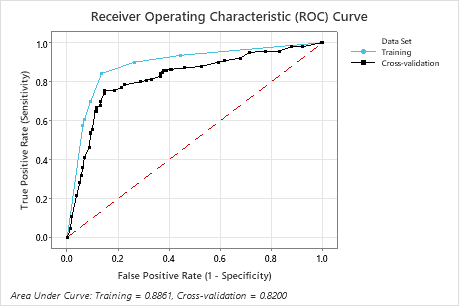# Receiver operating characteristic (ROC) curve for CART® Classification

The ROC curve plots the true positive rate (TPR), also known as power, on the y-axis. The ROC curve plots the false positive rate (FPR), also known as type 1 error, on the x-axis. The area under an ROC curve indicates whether the classification tree is a good classifier.

## Interpretation

For classification trees, the area under the ROC curve values range from 0.5 to 1. When a classification tree can perfectly separate the classes, then the area under the curve is 1. When a classification tree cannot separate the classes better than a random assignment, then the area under the curve is 0.5. The red dotted line indicates the random assignment case.In this example, the training and test curves are similar to each other. The area under the test curve is 0.820.
By using this site you agree to the use of cookies for analytics and personalized content.  Read our policy